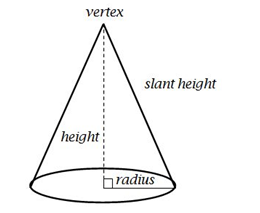Courses
Courses for Kids
Free study material
Free LIVE classes
MoreLIVE
Join Vedantu’s FREE Mastercalss

# Diameter of the base of a cone is 10.5cm and its slant height is 10cm. Find its curved surface area.Verified
363.6k+ views
Hint: Slant height of a cone is the line joining the vertex to any point on a circular edge of the base.

Now, they have given us the diameter of the base of a cone as $d = 10.5cm$
And, therefore, radius of the base of cone will be $r = 5.25cm$
And, the slant height given is $\ell = 10cm$The formula to find the curved surface area of the cone, $A = \pi r\ell$
We already have all the values, let us substitute it in the above formula,
We get,
$A = \dfrac{{22}}{7} \times 5.25 \times 10$
$A = 165c{m^2}$
Answer= the curved surface area of the given cone is n$165c{m^2}$
Note: Do not forget to convert the diameter to radius before taking the value or make sure that you take the correct formula. If you have been given the diameter, make sure to use the formula which has diameter or if they have given the radius, make sure to use formula which has radius in it and not the other way round.

Last updated date: 29th Sep 2023
Total views: 363.6k
Views today: 3.63k